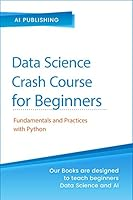# Statistics Crash Course for Beginners: Theory and Applications of Frequentist and Bayesian Statistics Using Python## Book Description

### Frequentist and Bayesian Statistics Crash Course for Beginners

Data and statistics are the core subjects of Machine Learning (ML). The reality is the average programmer may be tempted to view statistics with disinterest. But if you want to exploit the incredible power of Machine Learning, you need a thorough understanding of statistics. The reason is a Machine Learning professional develops intelligent and fast algorithms that learn from data.
Frequentist and Bayesian Statistics Crash Course for Beginners presents you with an easy way of learning statistics fast.
Contrary to popular belief, statistics is no longer the exclusive domain of math Ph.D.s. It’s true that statistics deals with numbers and percentages. Hence, the subject can be very dry and boring. This book, however, transforms statistics into a fun subject.
Frequentist and Bayesian statistics are two statistical techniques that interpret the concept of probability in different ways. Bayesian statistics was first introduced by Thomas Bayes in the 1770s.
Bayesian statistics has been instrumental in the design of high-end algorithms that make accurate predictions. So even after 250 years, the interest in Bayesian statistics has not faded. In fact, it has accelerated tremendously.
Frequentist Statistics is just as important as Bayesian Statistics. In the statistical universe, Frequentist Statistics is the most popular inferential technique. In fact, it’s the first school of thought you come across when you enter the statistics world.

### How Is This Book Different?

AI Publishing is completely sold on the learning by doing methodology. We have gone to great lengths to ensure you find learning statistics easy. The result: you will not get stuck along your learning journey.
This is not a book full of complex mathematical concepts and difficult equations. You will find that the coverage of the theoretical aspects of statistics is proportionate to the practical aspects of the subject.
The book makes the reading process easier by presenting you with three types of box-tags in different colors. They are: RequirementsFurther Readings, and Hands-on Time.
The final chapter presents two mini-projects to give you a better understanding of the concepts you studied in the previous eight chapters.
The main feature is you get instant access to a treasure trove of all the related learning material when you buy this book. They include PDFs, Python codes, exercises, and references—on the publisher’s website. You get access to all this learning material at no extra cost. You can also download the Machine Learning datasets used in this book at runtime. Alternatively, you can access them through the Resources/Datasets folder.
The quick course on Python programming in the first chapter will be immensely helpful, especially if you are new to Python. Since you can access all the Python codes and datasets, a computer with the internet is sufficient to get started.

The topics covered include:

• A Quick Introduction to Python for Statistics
• Starting with Probability
• Random Variables and Probability Distributions
• Descriptive Statistics: Measure of Central Tendency and Spread
• Exploratory Analysis: Data Visualization
• Statistical Inference
• Frequentist Inference
• Bayesian Inference
• Hands-on Projects

## Book Details

• Title: Statistics Crash Course for Beginners: Theory and Applications of Frequentist and Bayesian Statistics Using Python
• Author:
• Edition: 1
• Language: English
• Publisher:
• Publication Date: 2020-11-10
• ISBN-10: B08N8NSPPF